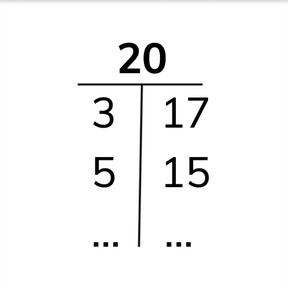Number bond charts to 20

# Number bond charts to 20

Number bond charts to 20

No account needed.8,000 schools use Gynzy92,000 teachers use Gynzy1,600,000 students use Gynzy

## General

Students practice decomposing numbers to 20 using and completing number bond charts.

## Relevance

It is useful to be able to decompose numbers if you want to divide objects into two groups. An example is if you are going to hand out books with another student. The one student gets 12 and the other 8. Ask students to come up with other examples.

## Introduction

Students practice composing numbers to 15 with blocks. For example, the first students sets down 13 blocks and the second student must compose 15 by adding 2 more blocks to the 13. They take turns.

## Development

Show that you can decompose numbers in different ways. Use the eggs to demonstrate decomposing the number 18. Show that you can turn that into 10 and 8, but also 12 and 6. This is called decomposing a number. If you already have one of the numbers, you simply count on to see how many are needed. Explain that that is called composing a number. Show the number bond chart of 12. Show all decompositions of 12 and say that you can always switch the numbers in a number bond. Students practice decomposing 13 and 14 by dragging objects on the board and completing the number bond charts. There are also decompositions where you only get a number without any objects. Ask students to do the decomposition in their heads, but allow them to use blocks as support. Ask them to complete the number bond chart for 16. Notice that the numbers are switched.

Check that students understand by asking the following questions:
- What does it mean to decompose a number?
- Can you name a decomposition (number bond) of 17?
- What is a number bond chart? (shows the decompositions of a number)

## Guided Practice

Students practice decomposition first by completing a number bond chart with objects as visual support and then move to completing a number bond chart with only the number given.

## Closing

Discuss why it is useful to be able to decompose numbers. You can divide objects into two groups in different ways. Check that students can complete the number bond charts. Use the exercise with cherries to help restate learning goals.

## Teaching Tip

Students who find this goal challenging can use manipulatives while completing the number bond charts to show their decompositions.

## Instruction materials

books, blocks

### The online teaching platform for interactive whiteboards and displays in schools

• Save time building lessons

• Manage the classroom more efficiently

• Increase student engagement